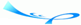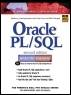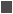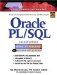# Lab 6.2 CASE Expressions

 Team-FlyOracle® PL/SQL® Interactive Workbook, Second EditionBy Benjamin Rosenzweig, Elena Silvestrova Table of Contents Chapter 6.  Conditional Control: Case Statements

Lab Objectives

After this Lab, you will be able to:

• Use CASE Expressions

In Chapter 3, you encountered various PL/SQL expressions. You will recall that the result of an expression yields a single value that is assigned to a variable. In a similar manner, a CASE expression evaluates to a single value that is then assigned to a variable.

A CASE expression has a structure almost identical to a CASE statement. Thus, it also has two forms: CASE and searched CASE. Consider an example of a CASE statement used in the previous lab of this chapter:FOR EXAMPLE

` DECLARE     v_num NUMBER := &sv_user_num;     v_num_flag NUMBER;  BEGIN     v_num_flag := MOD(v_num,2);     -- test if the number provided by the user is even     CASE v_num_flag        WHEN 0 THEN           DBMS_OUTPUT.PUT_LINE (v_num||' is even number');        ELSE           DBMS_OUTPUT.PUT_LINE (v_num||' is odd number');     END CASE;     DBMS_OUTPUT.PUT_LINE ('Done');  END; `

Consider the new version of the same example, with the CASE expression instead of the CASE statement:FOR EXAMPLE

` DECLARE     v_num NUMBER := &sv_user_num;     v_num_flag NUMBER;     v_result VARCHAR2(30);  BEGIN     v_num_flag := MOD(v_num,2);     v_result :=        CASE v_num_flag           WHEN 0 THEN v_num||' is even number'           ELSE v_num||' is odd number'        END;     DBMS_OUTPUT.PUT_LINE (v_result);     DBMS_OUTPUT.PUT_LINE ('Done');  END; `

In this example, a new variable, v_result, is used to hold the value returned by the CASE expression. If v_num is assigned the value of 8, this example produces the following output:

` Enter value for sv_user_num: 8  old   2:    v_num NUMBER := &sv_user_num;  new   2:    v_num NUMBER := 8;  8 is even number  Done  PL/SQL procedure successfully completed. `

It is important to note some syntax differences between a CASE statement and a CASE expression. Consider the following code fragments:

Case Statement

Case Expression

` CASE v_num_flag     WHEN 0 THEN        DBMS_OUTPUT.PUT_LINE           (v_num||' is even number');     ELSE        DBMS_OUTPUT.PUT_LINE        (v_num||' is odd number');  END CASE; `

` CASE v_num_flag     WHEN 0 THEN        v_num||        ' is even number'     ELSE        v_num||        ' is odd number'  END; `

In the CASE statement, the WHEN and ELSE clauses each contain a single executable statement. Each executable statement is terminated by a semicolon. In the CASE expression, the WHEN and ELSE clauses each contain an expression that is not terminated by a semicolon. There is one semicolon present after the reserved word END, which terminates the CASE expression. Finally, the CASE statement is terminated by the reserved phrase END CASE.

Next, consider another version of the previous example, with the searched CASE expression:FOR EXAMPLE

` DECLARE     v_num NUMBER := &sv_user_num;     v_result VARCHAR2(30);  BEGIN     v_result :=        CASE           WHEN MOD(v_num,2) = 0 THEN v_num||' is even number'           ELSE v_num||' is odd number'        END;     DBMS_OUTPUT.PUT_LINE (v_result);     DBMS_OUTPUT.PUT_LINE ('Done');  END; `

In this example, there is no need to declare variable v_num_flag because the searched CASE expression does not need a selector value, and the result of the MOD function is incorporated into the search condition. When run, this example produces output identical to the previous version:

` Enter value for sv_user_num: 8  old   2:    v_num NUMBER := &sv_user_num;  new   2:    v_num NUMBER := 8;  8 is even number  Done  PL/SQL procedure successfully completed. `

You learned earlier that a CASE expression returns a single value that is then assigned to a variable. In the examples that you saw earlier, this assignment operation was accomplished via the assignment operator, :=. You may recall that there is another way to assign a value to a PL/SQL variable, via a SELECT INTO statement. Consider an example of the CASE expression used in a SELECT INTO statement:FOR EXAMPLE

` DECLARE     v_course_no NUMBER;     v_description VARCHAR2(50);     v_prereq VARCHAR2(35);  BEGIN     SELECT course_no, description,            CASE               WHEN prerequisite IS NULL THEN                  'No prerequisite course required'               ELSE TO_CHAR(prerequisite)            END prerequisite       INTO v_course_no, v_description, v_prereq       FROM course      WHERE course_no = 20;     DBMS_OUTPUT.PUT_LINE ('Course: '||v_course_no);     DBMS_OUTPUT.PUT_LINE ('Description: '||v_description);     DBMS_OUTPUT.PUT_LINE ('Prerequisite: '||v_prereq);  END; `

In this example, you are displaying course number, description, and the number of a prerequisite course on the screen. Furthermore, if a given course does not have a prerequisite course, a message stating so is displayed on the screen. In order to achieve the desired results, a CASE expression is used as one of the columns in the SELECT INTO statement. Its value is assigned to the variable v_prereq. Notice that there is no semicolon after the reserved word END of the CASE expression.

This example produces the following output:

` Course: 20  Description: Intro to Computers  Prerequisite: No prerequisite course required  PL/SQL procedure successfully completed. `

Course 20 does not have a prerequisite course. As a result, the searched condition

` WHEN prerequisite IS NULL THEN `

evaluates to TRUE, and the value "No prerequisite course required" is assigned to the variable v_prereq.

It is important to note why function TO_CHAR is used in the ELSE clause of the CASE expression:

` CASE     WHEN prerequisite IS NULL THEN 'No prerequisite course     required'     ELSE TO_CHAR(prerequisite)  END `

A CASE expression returns a single value, thus, a single datatype. Therefore, it is important to ensure that regardless of what part of a CASE expression is executed, it always returns the same datatype. In the preceding CASE expression, the WHEN clause returns the VARCHAR2 datatype. The ELSE clause returns the value of the PREREQUISITE column of the COURSE table. This column has been defined as NUMBER, so it is necessary to convert it to the string datatype.

When the TO_CHAR function is not used, the CASE expression causes the following syntax error:

`              ELSE prerequisite                    *  ERROR at line 9:  ORA-06550: line 9, column 19:  PL/SQL: ORA-00932: inconsistent datatypes  ORA-06550: line 6, column 4:  PL/SQL: SQL Statement ignored `

#### Lab 6.2 Exercises

##### 6.2.1 Use the CASE Expression

In this exercise, you will modify the script ch06_2a.sql. Instead of using a searched CASE statement, you will use a searched CASE expression to display a letter grade for a student registered for a specific section of course number 25.

Answer the following questions:

 a) Modify the script ch06_2a.sql. Substitute the CASE statement with the searched CASE expression, and assign the value returned by the expression to the variable v_letter_grade. b) Run the script created in part a and explain the output produced. c) Rewrite the script created in part a so that the result of the CASE expression is assigned to the v_letter_grade variable via a SELECT INTO statement.

#### Lab 6.2 Exercise Answers

This section gives you some suggested answers to the questions in Lab 6.2, with discussion related to how those answers resulted. The most important thing to realize is whether your answer works. You should figure out the implications of the answers here and what the effects are from any different answers you may come up with.

##### 6.2.1 Answers

 a) Modify the script ch06_2a.sql. Substitute the CASE statement with the searched CASE expression, and assign the value returned by the expression to the variable v_letter_grade. A1: Answer: Your script should look similar to the script below. Changes are shown in bold letters.` -- ch06_3a.sql, version 1.0 SET SERVEROUTPUT ON DECLARE v_student_id NUMBER := 102; v_section_id NUMBER := 89; v_final_grade NUMBER; v_letter_grade CHAR(1); BEGIN SELECT final_grade INTO v_final_grade FROM enrollment WHERE student_id = v_student_id AND section_id = v_section_id; v_letter_grade := CASE WHEN v_final_grade >= 90 THEN 'A' WHEN v_final_grade >= 80 THEN 'B' WHEN v_final_grade >= 70 THEN 'C' WHEN v_final_grade >= 60 THEN 'D' ELSE 'F' END; -- control resumes here DBMS_OUTPUT.PUT_LINE ('Letter grade is: '|| v_letter_grade); END; `In the original version of the script (ch06_2a.sql), you used a searched CASE statement in order to assign a value to the variable v_letter_grade as follows:` CASE WHEN v_final_grade >= 90 THEN v_letter_grade := 'A'; WHEN v_final_grade >= 80 THEN v_letter_grade := 'B'; WHEN v_final_grade >= 70 THEN v_letter_grade := 'C'; WHEN v_final_grade >= 60 THEN v_letter_grade := 'D'; ELSE v_letter_grade := 'F'; END CASE; `Notice that the variable v_letter_grade was used as part of the CASE statement. In the new version of the script, the CASE expression ` CASE WHEN v_final_grade >= 90 THEN 'A' WHEN v_final_grade >= 80 THEN 'B' WHEN v_final_grade >= 70 THEN 'C' WHEN v_final_grade >= 60 THEN 'D' ELSE 'F' END; `does not contain any references to the variable v_letter_grade. Each search condition is evaluated. As soon as a particular condition evaluates to TRUE, its corresponding value is returned and then assigned to the variable v_letter_grade. b) Run the script created in part a and explain the output produced. A2: Answer: Your output should look similar to the following:` Letter grade is: A PL/SQL procedure successfully completed. `The SELECT INTO statement returns a value of 92 that is assigned to the variable v_final_grade. As a result, the first searched condition of the CASE expression evaluates to TRUE and returns a value of 'A'. This value is then assigned to the variable v_letter_grade and displayed on the screen via the DBMS_OUTPUT.PUT_LINE statement. c) Rewrite the script created in part a so that the result of the CASE expression is assigned to the v_letter_grade variable via a SELECT INTO statement. A3: Answer: Your script should look similar to the following. Changes are shown in bold letters.` -- ch06_3b.sql, version 2.0 SET SERVEROUTPUT ON DECLARE v_student_id NUMBER := 102; v_section_id NUMBER := 89; v_letter_grade CHAR(1); BEGIN SELECT CASE WHEN final_grade >= 90 THEN 'A' WHEN final_grade >= 80 THEN 'B' WHEN final_grade >= 70 THEN 'C' WHEN final_grade >= 60 THEN 'D' ELSE 'F' END INTO v_letter_grade FROM enrollment WHERE student_id = v_student_id AND section_id = v_section_id; -- control resumes here DBMS_OUTPUT.PUT_LINE ('Letter grade is: '|| v_letter_grade); END; `In the previous version of the script, the variable v_final_grade was used to hold the value of the numeric grade.` SELECT final_grade INTO v_final_grade FROM enrollment WHERE student_id = v_student_id AND section_id = v_section_id; `This value was used by the CASE expression to assign proper letter grade to the variable v_letter_grade.` CASE WHEN v_final_grade >= 90 THEN 'A' WHEN v_final_grade >= 80 THEN 'B' WHEN v_final_grade >= 70 THEN 'C' WHEN v_final_grade >= 60 THEN 'D' ELSE 'F' END; `In the current version of the script, the CASE expression is used as part of the SELECT INTO statement. As a result, the column FINAL_GRADE can be used by the CASE expression` CASE WHEN final_grade >= 90 THEN 'A' WHEN final_grade >= 80 THEN 'B' WHEN final_grade >= 70 THEN 'C' WHEN final_grade >= 60 THEN 'D' ELSE 'F' END `as part of the searched conditions in order to assign a value to the variable v_letter_grade.

#### Lab 6.2 Self-Review Questions

In order to test your progress, you should be able to answer the following questions.

Answers appear in Appendix A, Section 6.2.

 1) A CASE expression _____ returns a single value._____ returns multiple values._____ does not return values at all 2) A CASE expression is terminated by _____ END CASE._____ CASE._____ END. 3) A CASE expression never has a selector. _____ True_____ False 4) When all conditions of a CASE expression evaluate to NULL, the expression _____ returns NULL if there is no ELSE clause present._____ causes a syntax error if there is no ELSE clause present. 5) A CASE expression may return a single datatype only. _____ True_____ False

 Team-FlyTopOracle PL/SQL Interactive Workbook (2nd Edition)
ISBN: 0130473200
EAN: 2147483647
Year: 2002
Pages: 146

Similar book on Amazon

flylib.com © 2008-2017.
If you may any questions please contact us: flylib@qtcs.net